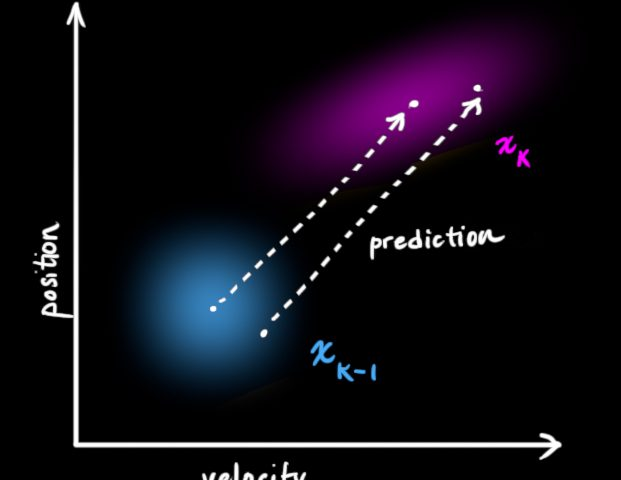April 26, 2020
###### Installing g++ (C++ Compiler) on Windows
May 9, 2020Let’s start from what a Kalman filter is: It’s a method of predicting the future state of a system based on the previous ones.

$\text{test} 2x \rightarrow$

To understand what it does, take a look at the following data – if you were given the data in blue, it may be reasonable to predict that the green dot should follow, by simply extrapolating the linear trend from the few previous samples. However, how confident would you be predicting the dark red point on the right using that method? how confident would you about predicting the green point, if you were given the red series instead of the blue?

From this simple example, we can learn three important principles:

1. It’s not good enough to give a prediction – you also want to know the confidence level.
2. Predicting far ahead into the future is less reliable than nearer predictions.
3. The reliability of your data (the noise), influences the reliability of your predictions.

⟵∽∗ ∗ ∗∼⟶⟵∽∗ ∗ ∗∼⟶

Now, let’s try and use the above to model our prediction.

The first thing we need is a state. The state is a description of all the parameters we will need to describe the current system and perform the prediction. For the example above, we’ll use two numbers: The current vertical position (yy), and our best estimate of the current slope (let’s call it mm). Thus, the state is in general a vector, commonly denoted xx, and you of course can include many more parameters to it if you wish to model more complex systems.

The next thing we need is a model: The model describes how we think the system behaves. In an ordinary Kalman filter, the model is always a linear function of the state. In our simple case, our model is:y(t)=y(t−1)+m(t−1)y(t)=y(t−1)+m(t−1)m(t)=m(t−1)m(t)=m(t−1)Expressed as a matrix, this is:xt=(y(t)m(t))=(1011)⋅(y(t−1)m(t−1))≡Fxt−1xt=(y(t)m(t))=(1101)⋅(y(t−1)m(t−1))≡Fxt−1

Of course, our model isn’t perfect (else we wouldn’t need a Kalman Filter!), so we add an additional term to the state – the process noise, vtvt which is assumed to be normally distributed. Although we don’t know the actual value of the noise, we assume we can estimate how “large” the noise is, as we shall presently see. All this gives us the state equation, which is simply:xt=Fxt−1+vt−1xt=Fxt−1+vt−1The third part, and final part we are missing is the measurement. When we get new data, our parameters should change slightly to refine our current model, and the next predictions. What is important to understand is that one does not have to measure exactly the same parameters as the those in the state. For instance, a Kalman filter describing the motion of a car may want to predict the car’s acceleration, velocity and position, but only measure say, the wheel angle and rotational velocity. In our example, we only “measure” the vertical position of the new points, not the slope. That is:measurement=(10)⋅(y(t)m(t))measurement=(10)⋅(y(t)m(t))In the more general case, we may have more than one measurement, so the measurement is a vector, denoted by zz. Also, the measurements themselves are noisy, so the general measurement equation takes the form:zt=Hxt+wtzt=Hxt+wtWhere ww is the measurement noise, and HH is in general a matrix with width of the number of state variables, and height of the number of measurement variables.⟵∽∗ ∗ ∗∼⟶⟵∽∗ ∗ ∗∼⟶

Now that we have understood what goes into modeling the system, we can now start with the prediction stage, the heart of the Kalman Filter.

Let’s start by assuming our model is perfect, with no noise. How will we predict what our state will be at time t+1t+1? Simple! It’s just our state equation:x^t+1=Fxtx^t+1=FxtWhat do we expect to measure? simply the measurement equation:z^t+1=Hx^t+1z^t+1=Hx^t+1Now, what do we actually measure? probably something a bit different:y≡zt+1−z^t+1≠0y≡zt+1−z^t+1≠0The difference yy (also called the innovation) represents how wrong our current estimation is – if everything was perfect, the difference would be zero! To incorporate this into our model, we add the innovation to our state equation, multiplied by a matrix factor that tells us how much the state should change based on this difference between the expected and actual measurements:x^t+1=Fxt+Wyx^t+1=Fxt+WyThe matrix WW is known as the Kalman gain, and it’s determination is where things get messy, but understanding why the prediction takes this form is the really important part. But before we get into the formula for WW, we should give thought about what it should look like:

• If the measurement noise is large, perhaps the error is only a artifact of the noise, and not “true” innovation. Thus, if the measurement noise is large, WW should be small.
• If the process noise is large, i.e., we expect the state to change quickly, we should take the innovation more seriously, since it’ plausible the state has actually changed.
• Adding these two together, we expect:W∼Process NoiseMeasurement NoiseW∼Process NoiseMeasurement Noise

⟵∽∗ ∗ ∗∼⟶⟵∽∗ ∗ ∗∼⟶

One way to evaluate uncertainty of a value is to look at its variance. The first variance we care about is the variance of our prediction of the state:Pt=Cov(x^t)Pt=Cov(x^t)

Just like with xtxt, we can derive PtPt from its previous state:Pt+1=Cov(x^t+1)=Cov(Fxt)=FCov(xt)F⊤=FPtF⊤Pt+1=Cov(x^t+1)=Cov(Fxt)=FCov(xt)F⊤=FPtF⊤

However, this assumes our process model is perfect and there’s nothing we couldn’t predict. But normally there are many unknowns that might be influencing our state (maybe there’s wind, friction etc.). We incorporate this as a covariance matrix QQ of process noise vtvt and prediction variance becomes:Pt+1=FPtF⊤+QPt+1=FPtF⊤+Q

The last source of noise in our system is the measurement. Following the same logic we obtain a covariance matrix for z^t+1z^t+1.St+1=Cov(z^t+1)Cov(Hx^t+1)=HCov(x^t+1)H⊤=HPt+1H⊤St+1=Cov(z^t+1)Cov(Hx^t+1)=HCov(x^t+1)H⊤=HPt+1H⊤

As before, remember that we said that our measurements ztzt have normally distributed noise wtwt. Let’s say that the covariance matrix which describes this noise is called RR. Add it to measurement covariance matrix:St+1=HPt+1H⊤+RSt+1=HPt+1H⊤+R

Finally we can obtain WW by looking at how two normally distributed states are combined (predicted and measured):W=Pt+1H⊤S−1t+1

https://math.stackexchange.com/questions/840662/an-explanation-of-the-kalman-filter

https://www.bzarg.com/p/how-a-kalman-filter-works-in-pictures/##### Amir Masoud Sefidian
Data Scientist, Researcher, Software Developer